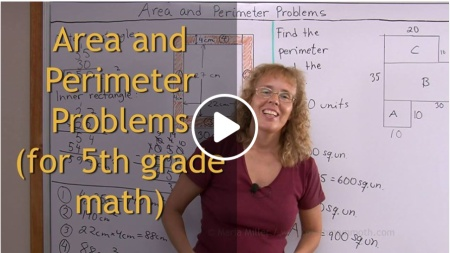^

# Free math video lessons for 5th gradeThis is a collection of free math videos for 5th grade, showing varied exercises for each topic. They match Math Mammoth Grade 5 curriculum but will also work no matter which curriculum you follow (in other words, the videos don't rely on you having Math Mammoth curriculum).

Please choose a topic from the list. I will expand the list as I'm able to edit and upload more videos.

## Chapter 1: The four operations

Review: Addition and Subtraction – this lesson uses bar models for addition and subtraction equations, plus teaches some math terms related to addition and subtraction

Review: Multiplication and Division – simple equations, bar models, terminology

Multiplying in Parts

The Multiplication Algorithm

The Multiplication Algorithm: 2-by-3 digit multiplication, plus a word problem

More Multiplication: 3-by-3 and 3-by-4 digit multiplications, multiplying numbers with lots of zeros, plus word problems

Divide in Parts (as part of the lesson Long Division in chapter 1)

Long division with a two-digit divisor

Long division as continued (repeated) subtraction

## Chapter 1: Number theory

Divisibility rules

(Introduction to primes — as a review from 4th grade)

Prime factorization using factor trees

## Chapter 2: Place value / large numbers

Exponents and powers

Rounding large numbers

Example: finding powers of 7 with a calculator

Examples of calculator usage: word problems

## Chapter 3: Problem solving

Balance problems and equations

Problem solving with bar models 1: fractional part of a whole

Problem solving with bar models 2: a word problem where total and difference are given

Problem solving with bar models 3

Problem solving with bar models 4

## Chapter 4: Decimals

Decimal place value and adding decimals, up to 2 decimal digits

Decimal place value (to thousandths)

Fractions to decimals, and comparing decimals — up to 3 decimal digits (thousandths)

Rounding decimals

Add & subtract decimals using mental math

Divide decimals by whole numbers (long division with decimals) — plus fractions to decimals

Divide decimals by whole numbers: problem solving

## Chapter 5: Graphing and Statistics

Coordinate grid

Numerical patterns in the coordinate grid

Line graphs

Drawing a histogram and a double bar graph

Mean and mode

## Chapter 6: Decimals

Multiplying decimals by decimals: the shortcut

Multiplying decimals and whole numbers: scaling

Divide decimals with mental math, part 1: sharing divisions (whole-number divisor)

Divide decimals with mental math, part 2: divisor is a decimal

Multiply and divide decimals by powers of ten

Divide decimals by decimals

Problem solving (with decimals)

Decimals in measuring units — introduction

The metric system (2 videos)

Rounding and estimating with measurements

## Chapter 7: Fractions: Add and Subtract

Mixed numbers to fractions and vice versa

Add mixed numbers (like fractional parts)

Subtract mixed numbers (like fractional parts)

Equivalent fractions

The least common denominator

Comparing fractions

## Chapter 8: Fractions: Multiply and Divide

Simplifying fractions

Multiply fractions by whole numbers

Multiply fractions by fractions

Fraction multiplication and area

Simplifying before multiplying

Multiplying mixed numbers

Multiplication as scaling/resizing

Fractions are divisions

Dividing fractions: sharing divisions

Dividing fractions: fitting the divisor

Dividing fractions: the shortcut

## Chapter 9: Geometry

Draw a square with a given side, and copy a triangle

Draw a hexagon and measure its angles

Circles

Classify triangles

Area and perimeter problems

Volume of a rectangular prism and cubic units

WAIT!

Receive my monthly collection of math tips & resources directly in your inbox — and get a FREE Math Mammoth book!You can unsubscribe at any time.

### Math Mammoth Tour

Confused about the different options? Take a virtual email tour around Math Mammoth! You'll receive:

An initial email to download your GIFT of over 400 free worksheets and sample pages from my books. Six other "TOURSTOP" emails that explain the important things and commonly asked questions concerning Math Mammoth curriculum. (Find out the differences between all these different-colored series!)

This way, you'll have time to digest the information over one or two weeks, plus an opportunity to ask me personally about the curriculum.
A monthly collection of math teaching tips & Math Mammoth updates (unsubscribe any time)### "Mini" Math Teaching Course

This is a little "virtual" 2-week course, where you will receive emails on important topics on teaching math, including:

- How to help a student who is behind
- Troubles with word problems
- Teaching multiplication tables
- Why fractions are so difficult
- The value of mistakes
- Should you use timed tests
- And more!

A GIFT of over 400 free worksheets and sample pages from my books right in the very beginning.A monthly collection of math teaching tips & Math Mammoth updates (unsubscribe any time)
Enter your email to receive math teaching tips, resources, Math Mammoth news & sales, humor, and more! I tend to send out these tips about once monthly, near the beginning of the month, but occasionally you may hear from me twice per month (and sometimes less often).• A GIFT of over 400 free worksheets and sample pages from my books.